﻿ 复杂波场页岩气探区速度分析方法探讨 A Discussion on Velocity Analysis Method of Shale Gas Exploration Area with Complex Wave Field

Journal of Oil and Gas Technology
Vol.40 No.06(2018), Article ID:28167,7 pages
10.12677/JOGT.2018.406117

A Discussion on Velocity Analysis Method of Shale Gas Exploration Area with Complex Wave Field

Yuan Du

Research Institution of Exploration and Development, East China Company, SINOPEC, Nanjing Jiangsu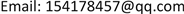Received: Jan. 8th, 2018; accepted: May 4th, 2018; published: Dec. 15th, 2018ABSTRACT

The wave field of XC Shale Gas Exploration Area was complex, and the stacking velocity was difficult to obtain. The velocity analysis by superposition energy method could not meet the processing requirements. This paper combined the characteristics of seismic data in the XC Shale Gas Exploration Area, focused on the impact of factors such as low signal-to-noise ratio and near-surface anomalies on the quality of the velocity spectrum, the targeted processing techniques and methods were proposed, a set of methods for precise extraction of seismic stack velocity in XC Shale Gas Exploration Area were summarized. Firstly, the original data were purified and the noise was suppressed by multi-domain and multi-method combination, and the signal-to-noise ratio was improved. Secondly, various residual static correction methods were used for maximally reducing the problem of high frequency static correction and improving the quality of seismic data. Finally, the relevant characteristics of instantaneous phase and the statistical phase correlation method were used to calculate the velocity spectrum, improve the precision of calculating speed. Better imaging results are obtained; especially the quality of structural imaging in the middle and deep layers is obviously improved.

Keywords:Complex Wave Field, Velocity Analysis, Phase Correlation, Velocity SpectrumXC页岩气探区波场复杂，叠加速度求取困难，采用叠加能量法进行速度分析不能满足处理要求。结合XC页岩气探区地震资料特点，重点针对信噪比低、近地表异常等因素对速度谱质量的影响，提出了针对性的技术，总结出一套精确提取XC页岩气探区地震叠加速度的方法。首先，对原始资料进行净化处理，采用多域、多方法组合压制噪音，提高信噪比；其次，应用多种剩余静校正法联合处理，最大程度减少高频静校正问题的存在，改善地震资料的品质；最后，利用瞬时相位的相关特性，应用统计相位相关法计算速度谱，提高速度求取的精度。该方法获得了较好的成像效果，特别是中深层的构造成像质量有明显改善。1. 引言

2. 速度分析基本原理

2.1. 叠加能量法

$A=\frac{1}{N}{\sum }_{j=1}^{M}\left({\sum }_{i-1}^{N}{f}_{i,j+{r}_{i}}\right)$ (1)

2.2. 统计相位相关法

1) 设输入的道集为uij，表示第i道、第j个采样点。设初始时间为t0，选定其对应的速度v进行动校正，此时可以得到校正后的道集，再应用Hilbert变换法求取uij的瞬时振幅Aij和瞬时相位μij

2) 将整个道集分为若干部分，即样点分布分为c组，样点的相位表示为 ${\mu }_{is}\left(i=1,2,\cdots ,{n}_{s};s=1,2,\cdots ,c\right)$ ，且 ${\sum }_{s=1}^{c}{n}_{s}=M$ ，求取统计量R和W：

$R=\sqrt{{\left({\sum }_{s=1}^{c}{U}_{s}\right)}^{2}+{\left({\sum }_{s=1}^{c}{W}_{s}\right)}^{2}}$ (2)

$W=2\left({\sum }_{s=1}^{c}\frac{{R}_{s}^{2}}{{n}_{s}}-\frac{{R}^{2}}{M}\right)$ (3)

R假设检验与W假设检验是判断t0及所选定的v正确与否的标准。当R检验假设概率分布是单峰分布时，代表t0及所选定的v正确；当W检验假设概率分布在不同偏移距范围内，且都是相同的单峰分布时，代表t0及所选定的v正确。

3) 计算常规叠加能量的瞬时信息SB(t, v)：

${S}_{\text{B}}\left(t,v\right)=\frac{{\sum }_{i=1}^{M}B\left[{\sum }_{j=1}^{N}{u}_{ij}\left(t,v\right)\right]}{{\sum }_{i=1}^{M}L\left\{{\sum }_{j=1}^{N}B\left[{u}_{ij}\left(t,v\right)\right]\right\}}$ (4)

4) 计算能量函数SRWB

${S}_{\text{RWB}}=\left(\frac{R}{W}\right){S}_{\text{B}}$ (5)

3. 应用实例效果分析

3.1. 速度分析的预处理

3.1.1. 提高信噪比处理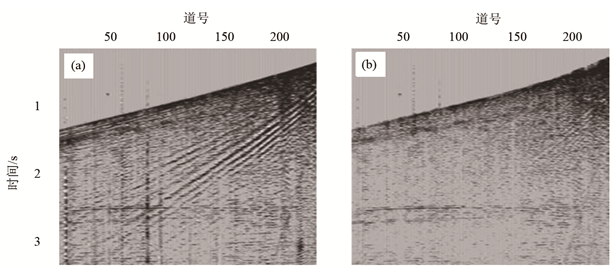Figure 1. The single shot result before (a) and after (b) denoising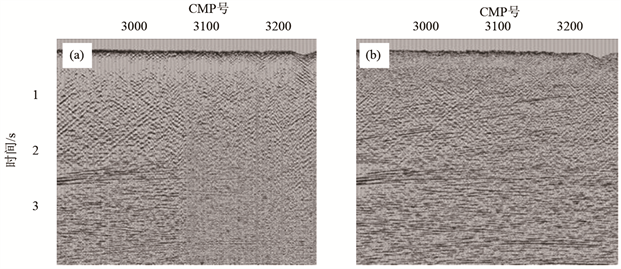Figure 2. The profile correlation before (a) and after (b) denoising

3.1.2. 剩余静校正处理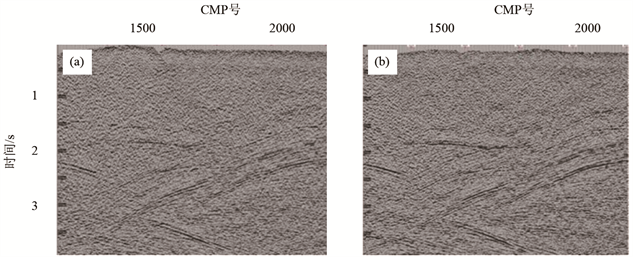Figure 3. The profile correlation before (a) and after (b) residual static calibration

3.2. 统计相位相关法速度谱的求取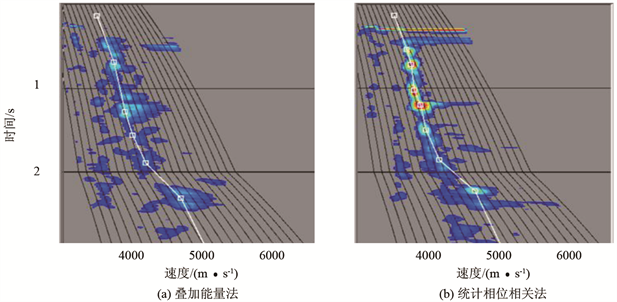Figure 4. The velocity spectrums of different velocity analysis methods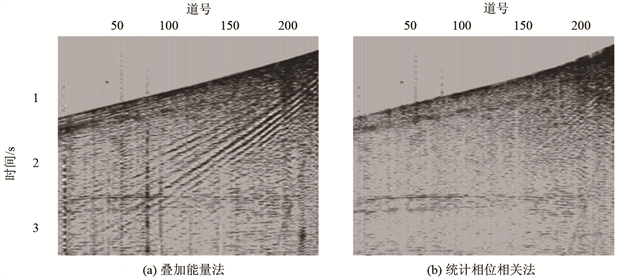Figure 5. The stack profiles of different velocity analysis methods

4. 结语

 李振春, 张军华. 地震数据处理方法[M]. 东营: 中国石油大学出版社, 2004: 1-308.

 李庆忠. 走向精确勘探的道路——高分辨率地震勘探系统工程剖析[M]. 北京: 石油工业出版社, 1993: 70-80.

 潘继平, 乔德武, 李世臻, 等. 下扬子地区古生界页岩气地质条件与勘探前景[J]. 地质通报, 2011, 30(2): 1671-2552.

 Turhan, T.M. and Koehler, F. (1969) Velocity Spectra-Digital Computer Derivation and Applications of Velocity Functions. Geophysics, 34, 859-881. https://doi.org/10.1190/1.1440058

 De Vries, D. and Berkhout, A.J. (1984) Velocity Analysis Based on Minimum Entropy. Geophysics, 49, 212-2142. https://doi.org/10.1190/1.1441629

 Biondi, B.L. and Kostov, C. (1989) High-Resolution Velocity Spectra Using Eigenstruture Methods. Geophysics, 54, 832-842. https://doi.org/10.1190/1.1442712

 Simon, K. (1991) Asymptotically Linear Velocity Analysis with High Resolution in Time Domain. Geophysics, 56, 1840-1848. https://doi.org/10.1190/1.1442997

 吴树初, 罗国安. 相关速度谱和复数道相关速度谱[J]. 石油地球物理勘探, 1990, 25(3): 333-341.

 林小竹. 协方差速度谱[J]. 石油地球物理勘探, 1993, 28(4): 403-410.

 Byun, B.S., Corrigan, D. and Gaiser, J.E. (1989) Anisotropic Velocity Analysis for Lithology Discrimination. Geophysics, 54, 1564-1574. https://doi.org/10.1190/1.1442624

 Alkhalifah, T. (1997) Velocity Analysis Using Nonhyperbolic Moveout in Transversely Isotropic Media. Geophysics, 62, 1839-1854. https://doi.org/10.1190/1.1444285

 Huub, D. and Alexander, C. (2006) Nonhyperbolic Moveout Analysis in VTI Media. Geophysics, 71, D59-D71. https://doi.org/10.1190/1.2194901

 Brahim, A., Bjorn, U. and Didier, R. (2009) Automatic Nonhyperbolic Velocity Analysis. Geophysics, 74, U1-U12.

 Sergey, F. and Alexey, S. (2010) Generalized Nonhyperbolic Moveout Approximation. Geophysics, 75, U9-U18. https://doi.org/10.1190/1.3334323

 徐翠娥, 郝晓红, 王影. 高密度双谱分析法在各向异性介质速度分析中的初步应用[J]. 海洋石油, 2008, 28(1): 1-5.

 Morozov, L.B. and Smithson, S.B. (1996) High-Resolution Velocity Determination: Statistical Phase Correlation and Image Processing. Geophysics, 61, 1115-1127. https://doi.org/10.1190/1.1444032

 王德利, 何樵登, 韩立国. 相位相关统计高分辨率速度分析[J]. 石油地球物理勘探, 2001, 36(2): 198-204.

 张军华, 王静, 郑旭刚, 等. 关于几种速度分析方法的讨论及效果分析[J]. 石油物探, 2009, 48(4): 347-353.

 谭胜章, 杜惠平, 宋国良, 等. 高精度三维地震资料采集技术[J]. 石油物探, 2007, 47(1): 74-80.

 王西文, 赵邦六. 地震资料采集方式对地震处理的影响研究[J]. 地球物理学进展, 2010, 25(3): 840-852.

 胡英, 张研, 陈立康, 等. 速度建模的影响因素与技术对策[J]. 石油物探, 2006, 45(5): 503-507.

 郭树祥. 高分辨率地震资料处理中的优化速度分析方法[J]. 石油物探, 2004, 43(1): 80-82.

 De Bazelaire, E. (1988) Nomudmoveou Revisited: Inhomogeneous Media and Curved Interfaces. Geophysics, 53, 143-157. https://doi.org/10.1190/1.1442449

[编辑] 龚丹